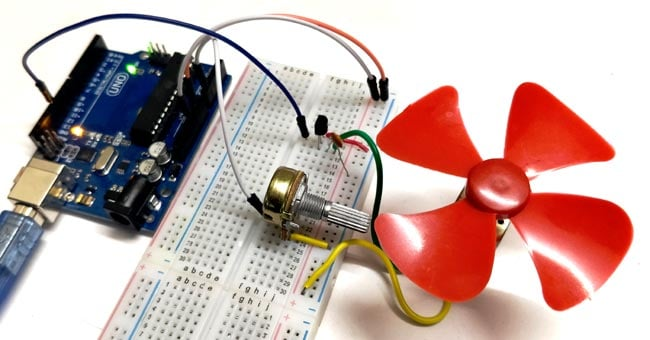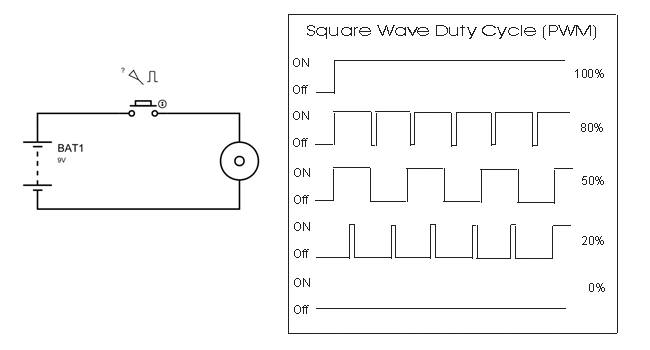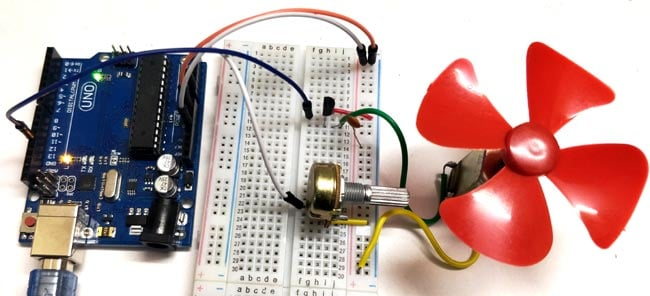# DC Motor Speed Control using Arduino and Potentiometer

Published  June 3, 2015   6DC motor is the most used motor in Robotics and electronics projects. For controlling the speed of DC motor we have various methods, like the speed can be automatically controlled based on temperature but in this project PWM method will be used to control the speed of DC motor. Here in this Arduino Motor Speed Control project, the speed can be controlled by rotating the knob of potentiometer.

### Pulse Width Modulation:

What is PWM? PWM is a technique by using we can control the voltage or power. To understand it more simply, if you are applying 5 volt for driving a motor then motor will moving with some speed, now if we reduces applied voltage by 2 means we apply 3 volt to motor then motor speed also decreases. This concept is used in the project to control the voltage using PWM. We have explained PWM in detail in this article. Also check this circuit where PWM is used to control the brightness of LED: 1 Watt LED Dimmer.

```% Duty cycle = (TON/(TON + TOFF)) *100

Where, TON = HIGH time of the square wave
TOFF = LOW time of square wave
```Now if the switch in the figure is closed continuously over a period of time then the motor will continuously ON during that time. If the switch is closed for 8ms and opened for 2ms over a cycle of 10ms, then the Motor will be ON only in the 8ms time. Now the average terminal over across the over a period of 10ms = Turn ON time/ (Turn ON time + Turn OFF time), this is called duty cycle and is of 80% (8/ (8+2)), so the average output voltage will be 80% of the battery voltage. Now human eye cannot see that motor is on for 8ms and off for 2ms, so will look like DC Motor is rotating with 80% speed.

In the second case, the switch is closed for 5ms and opened for 5ms over a period of 10ms, so the average terminal voltage at the output will be 50% of the battery voltage. Say if the battery voltage is 5V and the duty cycle is 50% and so the average terminal voltage will be 2.5V.

In the third case the duty cycle is 20% and the average terminal voltage is 20% of the battery voltage.

We have used PWM with Arduino in many of our Projects:

### Material Required

• Arduino UNO
• DC motor
• Transistor 2N2222
• Potentiometer 100k ohm
• Capacitor 0.1uF
• Jumping Wires

### Circuit Diagram

Circuit diagram for Arduino DC Motor Speed Control using PWM is geven below:

### Code and Explanation

The complete code for Arduino DC Motor Control using potentiometer is given at the end.

In the below code, we have initialized the variable c1 and c2 and assigned analog pin A0 for the potentiometer output and 12th Pin for ‘pwm’.

```int pwmPin = 12;
int pot = A0;
int c1 = 0;
int c2 = 0;  ```

Now, in the below code, setting pin A0 as input and 12 (which is PWM pin) as output.

```void setup()  {
pinMode(pwmPin, OUTPUT); // declares pin 12 as output
pinMode(pot, INPUT);  // declares pin A0 as input
}```

Now, in void loop (), we are reading the analog value (from A0) using analogRead(pot), and saving it to variable c2. Then, subtract c2 value from 1024 and save the result in c1. Then make the PWM pin 12th of Arduino HIGH and then after a delay of value c1 make that pin LOW. Again, after a delay of value c2 the loop continues.

The reason for subtracting Analog value from 1024 is, the Arduino Uno ADC is of 10-bit resolution (so the integer values from 0 - 2^10 = 1024 values). This means that it will map input voltages between 0 and 5 volts into integer values between 0 and 1024. So if we multiply input anlogValue to (5/1024), then we get the digital value of input voltage. Learn here how to use ADC input in Arduino

```void loop()
{
c1= 1024-c2;
digitalWrite(pwmPin, HIGH); // sets pin 12 HIGH
delayMicroseconds(c1);   // waits for c1 uS (high time)
digitalWrite(pwmPin, LOW);  // sets pin 12 LOW
delayMicroseconds(c2);   // waits for c2 uS (low time)
}```

### Speed Control of DC Motor using ArduinoIn this circuit, for controlling the speed of DC motor, we use a 100K ohm potentiometer to change the duty cycle of the PWM signal. 100K ohm potentiometer is connected to the analog input pin A0 of the Arduino UNO and the DC motor is connected to the 12th pin of the Arduino (which is the PWM pin). The working of Arduino program is very simple, as it reads the voltage from the analog pin A0. The voltage at analog pin is varied by using the potentiometer. After doing some necessary calculation the duty cycle is adjusted according to it.

For example, if we feed 256 value to the analog input, then the HIGH time will be 768ms (1024-256) and LOW time will be 256ms. Therefore, it simply means the duty cycle is 75%. Our eyes cannot see such high frequency oscillation and it looks like motor is continuously ON with 75% of speed. So that’s how we can perform Motor Speed Control using Arduino.

Code

int pwmPin = 12; // assigns pin 12 to variable pwm
int pot = A0; // assigns analog input A0 to variable pot
int c1 = 0;   // declares variable c1
int c2 = 0;   // declares variable c2

void setup()  // setup loop
{
pinMode(pwmPin, OUTPUT);
pinMode(pot, INPUT);
}

void loop()
{
c1= 1024-c2;         // subtracts c2 from 1000 ans saves the result in c1
digitalWrite(pwmPin, HIGH);
delayMicroseconds(c1);
digitalWrite(pwmPin, LOW);
delayMicroseconds(c2);
}

Video

Tags

### Excuse me, I'm worried about

Excuse me, I'm worried about test this circuit because, I've seen people damaging an Arduino for connecting it directly to a motor, and there are people that recommend to use an external power source for the motor. What is your opinion?

### Yes, there should be at…

Yes, there should be at least a flyback diode in parallel to the motor so that any induced voltage on the motor doesn't "hit" your circuit back.

### Hi, what is the purpose of…

Hi, what is the purpose of the capacitor and transistor? Why is it in this circuit? My thought is an amplifier. Is that correct?

### The capacitor is there to…

The capacitor is there to lower the EMI i think, while the transistor is used to control the average voltage to the motor. Think of it as a switch you switch on and off at a high freqency (PWM). For example, if the duty cycle is 50%, that means the transistor is conducting half of the period and not conducting the other half. The average voltage is therefore half of the max. PWM duty cycle here is controlled by the potentiometer as described.

### Please can you do the FIR…

Please can you do the FIR and IIR filter for this project to reduce the noise